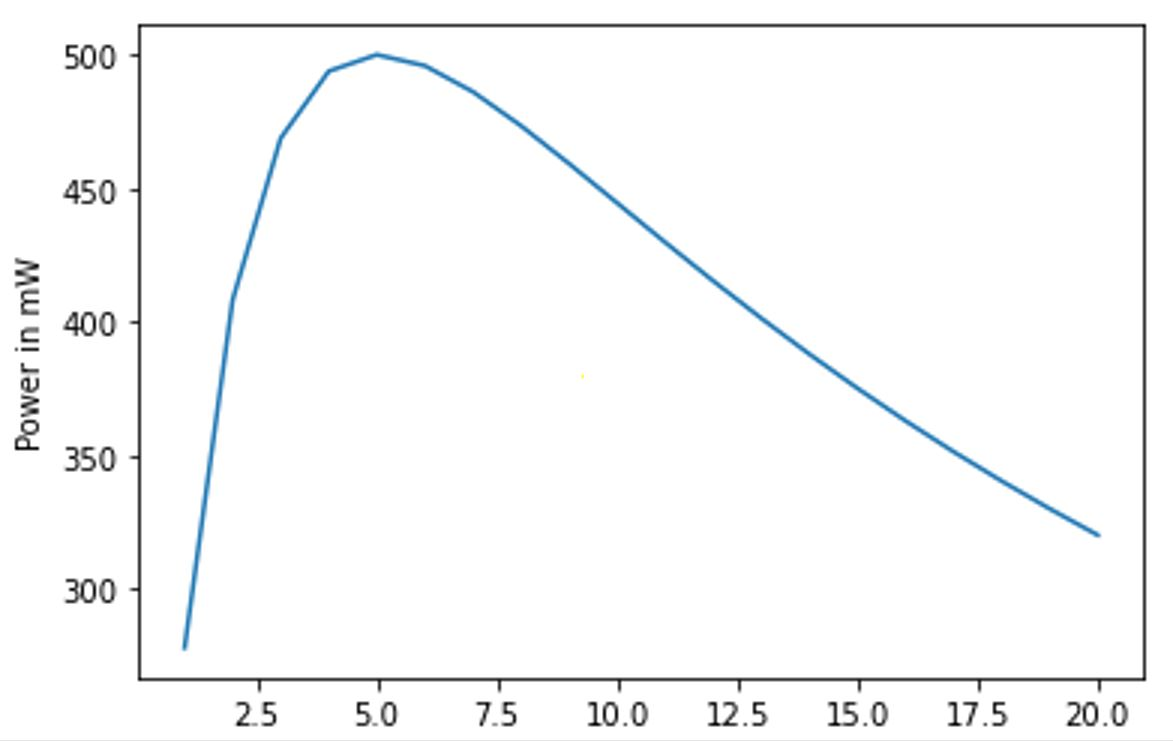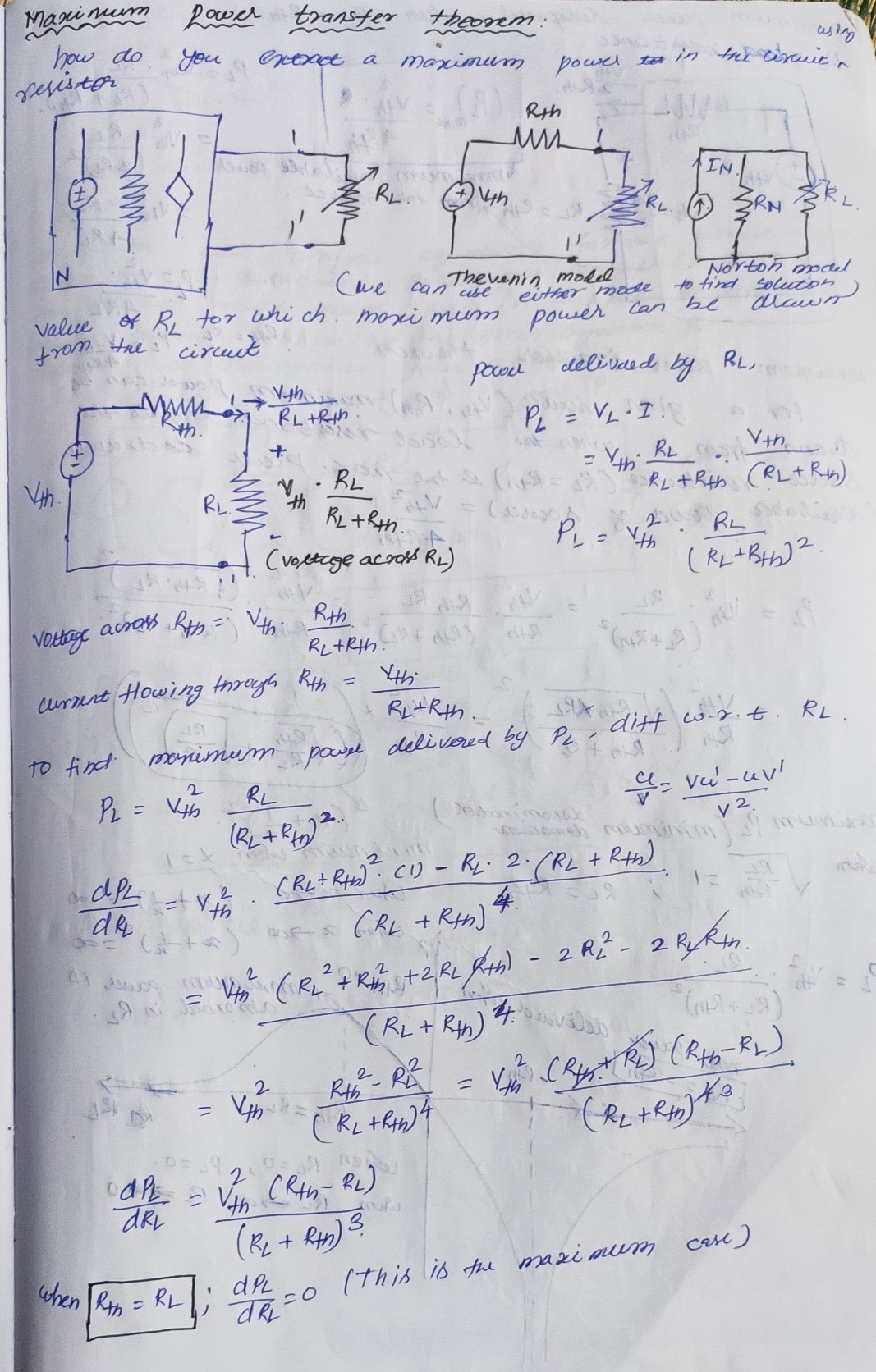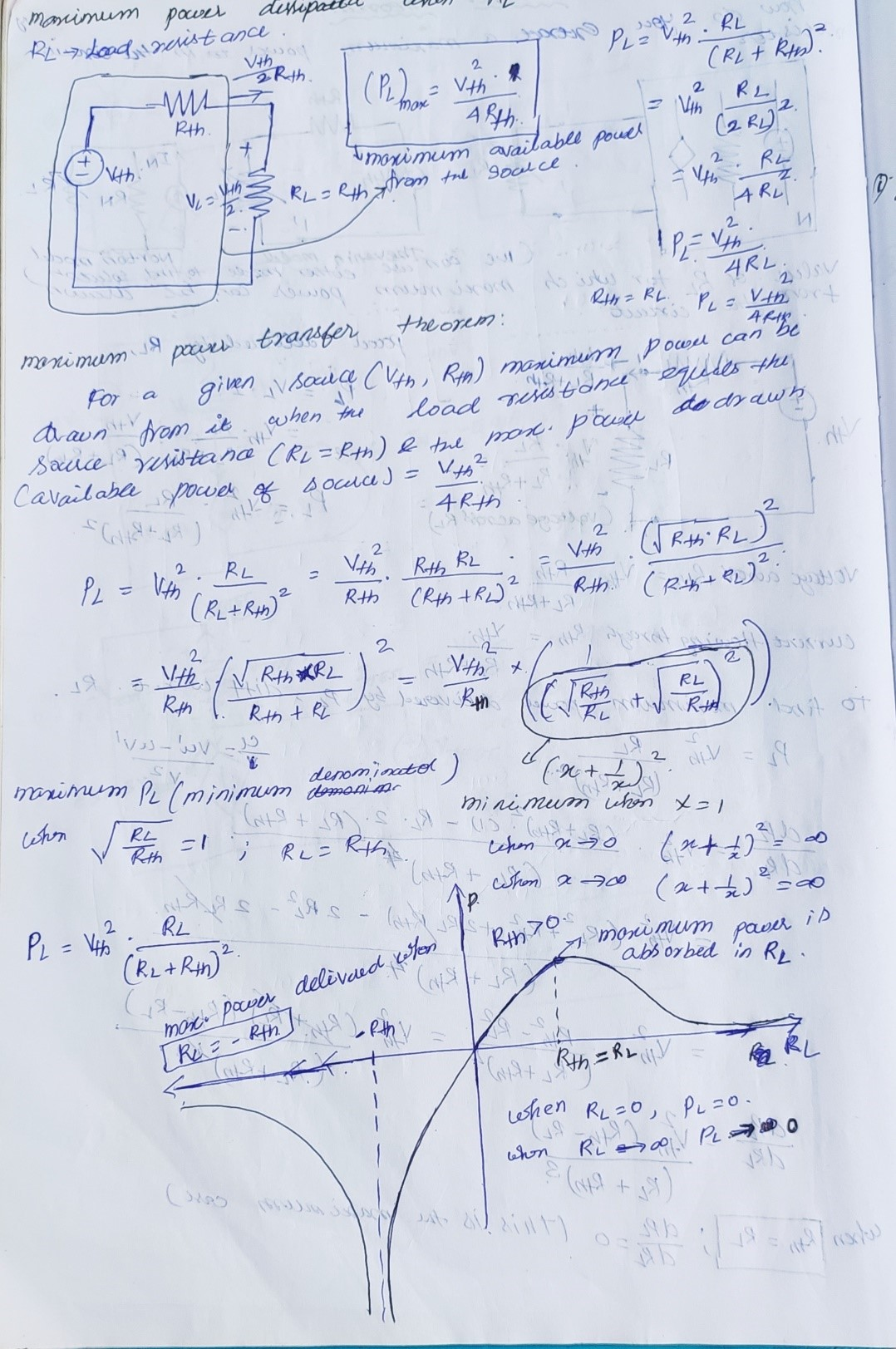# Maximum Power Transfer Theorem

I am doing Prof.Nagendra Krishna Pura’s Basics electrical circuits course provided by NPTEL. In week 6, I learnt about maximum power transfer theorem.

states that – A resistive load, being connected to a DC network, receives maximum power when the load resistance is equal to the internal resistance known as (Thevenin’s equivalent resistance) of the source network as seen from the load terminals. The Maximum Power Transfer theorem is used to find the load resistance for which there would be the maximum amount of power transfer from the source to the load.

To verify this theorem, I have taken Vth as 10V and Rth as 5K ohms connected in series with the load resistance RL. Now I am varying the RL to get the maximum powerFor doing this I have written a python program and plotted the graph power(mW) vs RL(Kohms)

# Maximum power transfer thorem using pyplot

from matplotlib import pyplot as plt

Rth = 5e3

Vth = 10

RL = [x*1e3 for x in range(21) if x >0]

Vth_across_RL = [res/(Rth+res) for res in RL ]

I = [Vth*1e3/(Rth+res) for res in RL]

def power(Vtholtage_list,current_list):

power_list = []

for i in range(len(Vtholtage_list)):

p = Vtholtage_list[i]*current_list[i]

power_list.append(p)

return power_list

#print(power(Vth_across_RL, I))

power_list =[x*1e3  for x in power(Vth_across_RL, I)]

RL_kohms = [x/1e3 for x in RL]

plt.plot(RL_kohms,power_list)

plt.xlabel(“Resistance in Kohms”)

plt.ylabel(“Power in mW”)

plt.show()From the graph I observed that when the load resistance (RL) is equal to the Thevenin resistance (Rth) of the circuits then I able to draw the maximum power from the source.